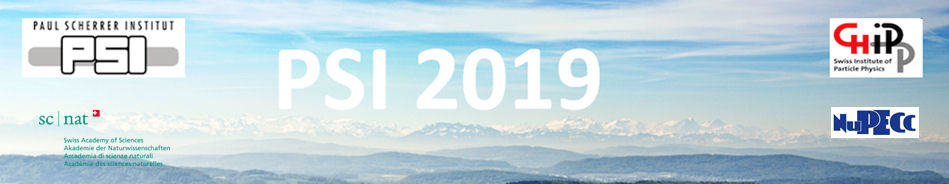#Physics of fundamental Symmetries and Interactions - PSI2019

Oct 20 – 25, 2019
PSI
Europe/Zurich timezone

## $g$-factor of Boronlike Argon 40 Ar13+ at ALPHATRAP

Oct 22, 2019, 3:30 PM
20m
WHGA/001 - Auditorium (PSI)

Oral

### Speaker

Ioanna Arapoglou (Max Planck Institute for Nuclear Physics, Saupfercheckweg 1, 69117 Heidelberg, Germany)

### Description

The ALPHATRAP $g$-factor experiment is a high-precision Penning-trap experiment located at the Max Planck Institute for Nuclear Physics in Heidelberg. It has been designed for performing high-precision $g$-factor measurements on heavy highly charged ions (HCI), such as hydrogenlike $^{208}\mathrm{Pb}^{81+}$ and boronlike $^{208}\mathrm{Pb}^{77+}$, which will be externally produced and injected into our setup. By determining the bound electron's $g$-factor, stringent test of bound-state quantum electrodynamics (BS-QED) can be carried out. Furthermore, such measurements can provide access to fundamental constants, such as the fine structure constant $\alpha$ which can be determined via the difference between the $g$-factor of a hydrogenlike and a boronlike ion. ALPHATRAP has been fully assembled and commissioned, after which the first measurement of the ground-state $g$-factor of boronlike argon $^{40}\mathrm{Ar}^{13+}$ took place. This measurement was performed with a fractional uncertainty of $1.4\times 10^{-9}$ which is almost 3 orders of magnitude more precise than the currently most precise theoretical prediction. Due to the optimised design, prominent systematic effects in predecessor experiments are highly suppressed in our setup. This experimental result constitutes the first high-precision $g$-factor determination of a boronlike ion as well as the first result of ALPHATRAP. Our experimentally determined $g$-factor of $^{40}\mathrm{Ar}^{13+}$ distinguishes between existing theoretical predictions that are in disagreement. Furthermore, this result lays the groundwork for BS-QED tests in even stronger fields and for the independent determination of the fine structure constant $\alpha$.

### Primary author

Ioanna Arapoglou (Max Planck Institute for Nuclear Physics, Saupfercheckweg 1, 69117 Heidelberg, Germany)

### Co-authors

Alexander Egl (Max Planck Institute for Nuclear Physics, Saupfercheckweg 1, 69117 Heidelberg, Germany) Martin H\"ocker (Max Planck Institute for Nuclear Physics, Saupfercheckweg 1, 69117 Heidelberg, Germany) Tim Sailer (Max Planck Institute for Nuclear Physics, Saupfercheckweg 1, 69117 Heidelberg, Germany) Robert Wolf (Max Planck Institute for Nuclear Physics, Saupfercheckweg 1, 69117 Heidelberg, Germany) Andreas Weigel (Max Planck Institute for Nuclear Physics, Saupfercheckweg 1, 69117 Heidelberg, Germany) Bingsheng Tu (Max Planck Institute for Nuclear Physics, Saupfercheckweg 1, 69117 Heidelberg, Germany) Sven Sturm (Max Planck Institute for Nuclear Physics, Saupfercheckweg 1, 69117 Heidelberg, Germany) Klaus Blaum (Max Planck Institute for Nuclear Physics, Saupfercheckweg 1, 69117 Heidelberg, Germany)